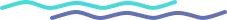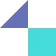# Exploring Perceptrons: The Building Blocks of Neural Networks

### Introduction

Perceptrons are a type of artificial neural network that are often considered the simplest form of a machine learning model.

They consist of a single layer of "neurons" (also known as computational units), which process input data and make predictions based on that data.

Perceptrons are typically used to solve classification problems, where the goal is to predict which of several possible classes an input data point belongs to.

Perceptrons are important because they are the building blocks of more complex neural networks.

By understanding how perceptrons work, we can gain insight into the fundamental mechanisms of neural networks and how they can be used to solve a wide range of problems in artificial intelligence. Additionally, the simplicity of perceptrons makes them a useful tool for learning about and experimenting with machine learning algorithms.

### History of Perceptrons

Perceptrons were first proposed by psychologist Frank Rosenblatt in the 1950s as a way to model the behavior of neurons in the human brain.

Rosenblatt developed a simple mathematical model of a single neuron, which could take in multiple inputs and produce a single output based on those inputs. This model formed the basis for the first perceptron algorithms, which were designed to mimic the basic functioning of the brain.

Over time, perceptrons have evolved and become more sophisticated. Today, they are often used as the building blocks of larger and more complex neural networks, which can tackle a wider range of problems in artificial intelligence. Researchers are continuing to explore new ways to improve the performance and capabilities of perceptrons, leading to exciting new developments in the field.

### How Perceptrons work

At its core, a perceptron consists of a single layer of computational units, or "neurons," which process input data and make predictions based on that data.

Each neuron receives a number of inputs, which are multiplied by a set of weights that represent the importance of each input to the neuron's prediction.

The neuron then applies a simple mathematical function, known as an activation function, to the weighted sum of its inputs to produce a single output.

For example, imagine we have a perceptron that is trying to predict whether a fruit is an apple or an orange based on its weight and color. The perceptron might have two inputs (one for weight and one for color), and two corresponding weights (one for each input). If an apple weighs more and is red, it might receive a higher weighted sum from the perceptron than an orange, indicating that the perceptron predicts it to be an apple.

Mathematically, we can represent a perceptron using the following equation:

output = activation_function(w1* x1 + w2 * x2 + ... + wn * xn + b)

Here,

a. x1 to xn represent the input values,

b. w1 to wn are the weights assigned to each input,

c. b is the bias term, and

d. activation_function is the activation function used by the perceptron.

The activation function determines whether the perceptron "fires" or not, based on the input it receives.

For example, the most common activation function used in perceptrons is the binary step function, which returns either 0 or 1 depending on whether the input is below or above a certain threshold value.

To see how this works in practice, let's consider a simple example where a perceptron takes in two inputs, x1 and x2, and has weights w1 and w2 assigned to them.

Let's say x1 and x2 are both equal to 1, and the weights are 0.5 and 0.3, respectively. The bias term b is set to -0.2. Using the equation above, we can calculate the output of the perceptron as follows:

output = activation_function(0.5 *1 +0.3 *1 -0.2)= activation_function(0.8)

If we assume the activation function is the binary step function, with a threshold of 0, then the output of the perceptron would be 1, since the input value of 0.8 is above the threshold.

If the threshold were set to a higher value, say 0.9, then the output of the perceptron would be 0, since the input value of 0.8 is below the threshold in this case.

By applying this simple process repeatedly across all of its neurons, a perceptron can make predictions for a wide range of classification problems. Because they are so simple, perceptrons are often used as a starting point for more complex neural network models.

### Real World Applications of Perceptrons

Perceptrons have a wide range of applications, from image recognition to natural language processing.

#### Image Recognition

A perceptron might be trained to identify objects in an image by analyzing the pixels and determining which ones are most important for making a prediction.

#### Natural Language Processing

In natural language processing, a perceptron might be used to analyze the words in a sentence and determine the grammatical role of each word.

#### Finance

Perceptrons are also used in finance, where they can be trained to identify patterns in stock market data and make predictions about future trends.

#### Healthcare

In healthcare, perceptrons can be used to analyze medical images and diagnose diseases, such as cancer.

#### Robotics

Perceptrons can be used to control the movement of robots and enable them to interact with their environment.

Overall, perceptrons are an important tool for solving many real-world problems in artificial intelligence. As the field continues to advance, we can expect to see even more exciting applications of perceptrons in the future.

### Trends shaping the future of perceptrons

One trend that is already starting to emerge is the use of more complex perceptron architectures, such as multi-layer perceptrons and convolutional neural networks. These architectures allow for the integration of multiple layers of neurons, which can provide greater predictive power and enable perceptrons to tackle more complex problems.

Another trend is the integration of perceptrons into larger and more sophisticated neural networks. By combining multiple perceptrons into a single network, researchers can build models that can solve a wider range of problems and achieve higher levels of accuracy.

Overall, the future of perceptrons is bright, and we can expect to see many exciting developments in the field in the coming years.

As the capabilities of perceptrons continue to improve, they will become an increasingly valuable tool for solving complex problems in artificial intelligence.

Tooliqa specializes in AI, Computer Vision, Deep Learning and Product Design UX/UI to help businesses simplify and automate their processes with our strong team of experts across various domains.

Want to know more on how AI can result in business process improvement? Let our experts guide you.

Reach out to us at business@tooli.qa.

## FAQs

Quick queries for this insight

What is a perceptron?A perceptron is the simplest type of artificial neural network, consisting of a single layer of computational units that process input data and make predictions based on that data. Perceptrons are typically used to solve classification problems, where the goal is to predict which of several possible classes an input data point belongs to.

How do perceptrons work?Perceptrons work by receiving multiple inputs, which are multiplied by a set of weights that represent the importance of each input to the perceptron's prediction. The perceptron then applies a simple mathematical function, known as an activation function, to the weighted sum of its inputs to produce a single output. By applying this process repeatedly across all of its neurons, a perceptron can make predictions for a wide range of classification problems.

Why are perceptrons important?Perceptrons are important because they are the building blocks of more complex neural networks. By understanding how perceptrons work, we can gain insight into the fundamental mechanisms of neural networks and how they can be used to solve a wide range of problems in artificial intelligence. Additionally, the simplicity of perceptrons makes them a useful tool for learning about and experimenting with machine learning algorithms.

### Connect with our experts today for a free consultation.

Oops! Something went wrong while submitting the form.

## Subscribe to Tooliqa

Oops! Something went wrong while submitting the form.## Built for Innovators

DICEWith advanced-tech offerings designed to handle challenges at scale, Tooliqa delivers solid infrastructure and solutioning which are built for to meet most difficult enterprise-level needs.​